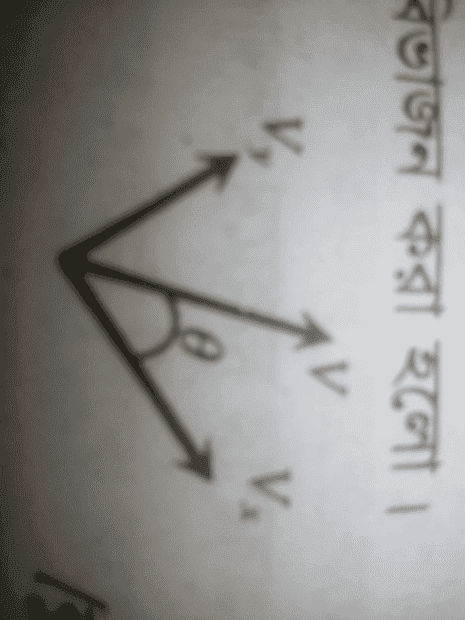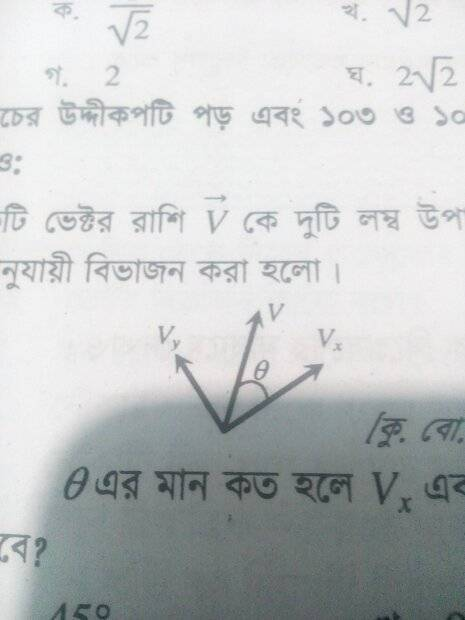# Find the value of ##\theta## when the Vx and Vy components are the same

Istiak
Homework Statement:
Find value of ##\theta## when ##V_x=V_y##
Relevant Equations:
VectorThe picture may be blurry. I couldn't take more less blurry picture hence, giving it.

The question is : Find value of ##\theta## when ##V_x## component and ##V_y## component same.

I was using a simple equation of vector.

$$C=\sqrt{A^2+B^2+2AB\cos\theta}$$
$$V=\sqrt{V_x^2+V_y^2+2V_xV_y\cos\theta}$$
$$=\sqrt{2V_x^2+2V_x^2\cos\theta}$$
$$=\sqrt{2V_x^2(1+\cos\theta)}$$
$$=V_x\sqrt{2 \cdot 2\cos^2(\frac{\theta}{2})}$$
$$=2V_x\cos\frac{\theta}{2}$$
$$2\cos^{-1}\frac{V}{2V_x}=\theta$$
Somehow, I took ##V=V_x## cause, I couldn't get any other way to remove them. When I took ##V=V_x## then, I got ##\theta=120 \deg##. But, which was completely wrong. The answer was ##45 \deg##. I was trying to use another equation. ##\vec A \cdot \vec B=AB\cos\theta##; Using the equation I got ##0## which is 100% wrong.

Homework Helper
Your $$V=\sqrt{V_x^2+V_y^2+2V_xV_y\cos\theta}$$ is a formula to calculate the length of ##\vec V## as the sum of ##\vec V_x## and ##\vec V_y## when the angle between ##\vec V_x## and ##\vec V_y## is ##\theta##. That angle is ##\ \pi/2\ ##, so the result is $$V=\sqrt{V_x^2+V_y^2}$$from which you can derive ##V## in terms of ##V_x##.

Your other equation $$\vec A \cdot \vec B=AB\cos\theta$$can then be used to find the angle between ##\vec V## and ##\vec V_x##

##\ ##

Istiak
Your $$V=\sqrt{V_x^2+V_y^2+2V_xV_y\cos\theta}$$ is a formula to calculate the length of ##\vec V## as the sum of ##\vec V_x## and ##\vec V_y## when the angle between ##\vec V_x## and ##\vec V_y## is ##\theta##. That angle is ##\ \pi/2\ ##, so the result is $$V=\sqrt{V_x^2+V_y^2}$$from which you can derive ##V## in terms of ##V_x##.

Your other equation $$\vec A \cdot \vec B=AB\cos\theta$$can then be used to find the angle between ##\vec V## and ##\vec V_x##

##\ ##
I wrote that ##\vec A \cdot \vec B = AB\cos\theta##
$$=>\vec A^2=A^2\cos\theta$$
$$=>1=\cos\theta$$
$$\theta=0$$
I am sure that I am mistaking with ##\cdot## I was getting more better way to multiply them. That's why I just wrote ##\vec A^2##. And, somehow I canceled vector A with A which was wrong either. So, how can I use the equation?

Homework Helper
Gold Member
2022 Award
The question is : Find value of ##\theta## when ##V_x## component and ##V_y## component same.
##tan\theta = \frac {V_y}{V_x}##

Edit: If the x and y are the other way round (picture too blurry to be sure) then ##tan\theta = \frac {V_x}{V_y}##.

•Istiak
Homework Helper
I wrote that $$\vec A \cdot \vec B = AB\cos\theta \Rightarrow \vec A^2=A^2\cos\theta$$ $$\Rightarrow 1=\cos⁡\theta \Rightarrow ⁡\theta =0$$
Which is correct: the angle between a vector and itself is zero.

I am sure that I am mistaking with ⋅ I was getting more better way to multiply them. That's why I just wrote A→2. And, somehow I canceled vector A with A which was wrong either. So, how can I use the equation?
Did you solve
That angle is ##\pi/2## , so the result is ##V=\sqrt { V_x^2+V_y^2} \ ## from which you can derive ##V## in terms of ##V_x##.
and what did you get ?

And what do you then get for ##\vec V \cdot \vec V_x\ ## ?

Of course, @Steve4Physics is showing you a direct short track to the answer.

##\ ##

IstiakYour $$V=\sqrt{V_x^2+V_y^2+2V_xV_y\cos\theta}$$ is a formula to calculate the length of ##\vec V## as the sum of ##\vec V_x## and ##\vec V_y## when the angle between ##\vec V_x## and ##\vec V_y## is ##\theta##. That angle is ##\ \pi/2\ ##, so the result is $$V=\sqrt{V_x^2+V_y^2}$$from which you can derive ##V## in terms of ##V_x##.

Your other equation $$\vec A \cdot \vec B=AB\cos\theta$$can then be used to find the angle between ##\vec V## and ##\vec V_x##

##\ ##
I got that $$V=\sqrt{2}V_x$$
so, when i put it in my main equation. I get $$2\cos^{-1}\sqrt{2}=\theta$$. But, cos inverse 1 of square root 2 is undefined.

Homework Helper
##V=\sqrt{2}V_x## is correct.

when i put it in my main equation
Your main equation being which one ? Is it applicable ?

Your other equation ##\vec A\cdot \vec B = A B\cos \theta \ ## can then be used to find the angle between ##\vec V## and ##\vec V_x##

And what do you then get for ## \vec V \cdot \vec V_x\ ## ?
You did not answer that. Why not ?

##\ ##

Istiak
Your main equation being which one ? Is it applicable ?
Forgot what you said... :)
You did not answer that. Why not ?
I was in mobile when writing my last reply. Umm! ##\vec V \cdot \vec V_x =V V_x \cos\theta##
$$=>\sqrt{2}\vec V_x \cdot \vec V_x=\sqrt{2} V_x^2 \cos\theta$$
$$=>\cos\theta=1$$

Blah! Still not working. I think I had missed LHS.

Which is correct: the angle between a vector and itself is zero.
Isn't that unit vector? ##\hat x=\frac{\vec x}{|x|}##

Homework Helper
$$=>\sqrt{2}\vec V_x \cdot \vec V_x$$ NOOOOO !You want to review the inner product !

Let ##\ \vec V = (V_x, V_y)\ ## and ##\ \vec V_x = (V_x, 0)\ ##
What is ##\vec V\cdot \vec V_x\ ## ?

##\ ##

Last edited:
Istiak
What is ##\vec V\cdot \vec V_x\ ## ?
##V V_x \cos\theta##

Homework Helper
Gold Member
2022 Award
View attachment 288322

I got that $$V=\sqrt{2}V_x$$
so, when i put it in my main equation. I get $$2\cos^{-1}\sqrt{2}=\theta$$. But, cos inverse 1 of square root 2 is undefined.
I assume you mean you plugged it into this in post #1:
##2\cos^{-1}\frac{V}{2V_x}=\theta##
But you made a mistake in the algebra. If you do it correctly you will get the answer.

•Istiak
Istiak
I assume you mean you plugged it into this in post #1:
##2\cos^{-1}\frac{V}{2V_x}=\theta##
But you made a mistake in the algebra. If you do it correctly you will get the answer.
I found where the mistake is. Although, I got ##90 \deg## instead of ##45 \deg##. If there was no constant ##2## outside of cosine then, I would get ##45 \deg##. But, it wasn't accurate way to remove ##2##. According #2 the equation won't work here.